# Work, Power, Potential Energy and Kinetic Energy Relations (Part - 1) Civil Engineering (CE) Notes | EduRev

## Civil Engineering (CE) : Work, Power, Potential Energy and Kinetic Energy Relations (Part - 1) Civil Engineering (CE) Notes | EduRev

The document Work, Power, Potential Energy and Kinetic Energy Relations (Part - 1) Civil Engineering (CE) Notes | EduRev is a part of the Civil Engineering (CE) Course Introduction to Dynamics and Vibrations- Notes, Videos, MCQs.
All you need of Civil Engineering (CE) at this link: Civil Engineering (CE)

In this chapter, we shall introduce (but not in this order) the following general concepts:

1. The linear impulse of a force
2. The angular impulse of a force
3. The power transmitted by a force
4. The work done by a force
5. The potential energy of a force.
6. The linear momentum of a particle (or system of particles)
7. The angular momentum of a particle, or system of particles.
8. The kinetic energy of a particle, or system of particles
9. The linear impulse momentum relations for a particle, and conservation of linear momentum
10. The principle of conservation of angular momentum for a particle
11. The principle of conservation of energy for a particle or system of particles.

We will also illustrate how these concepts can be used in engineering calculations. As you will see, to applying these principles to engineering calculations you will need two things: (i) a thorough understanding of the principles themselves; and (ii) Physical insight into how engineering systems behave, so you can see how to apply the theory to practice. The first is easy. The second is hard, and people who can do this best make the best engineers. 4.1 Work, Power, Potential Energy and Kinetic Energy relations The concepts of work, power and energy are among the most powerful ideas in the physical sciences. Their most important application is in the field of thermodynamics, which describes the exchange of energy between interacting systems. In addition, concepts of energy carry over to relativistic systems and quantum mechanics, where the classical versions of Newton’s laws themselves no longer apply. In this section, we develop the basic definitions of mechanical work and energy, and show how they can be used to analyze motion of dynamical systems. Future courses will expand on these concepts further.

4.1 Work, Power, Potential Energy and Kinetic Energy relations

The concepts of work, power and energy are among the most powerful ideas in the physical sciences. Their most important application is in the field of thermodynamics, which describes the exchange of energy between interacting systems. In addition, concepts of energy carry over to relativistic systems and quantum mechanics, where the classical versions of Newton’s laws themselves no longer apply.

In this section, we develop the basic definitions of mechanical work and energy, and show how they can be used to analyze motion of dynamical systems. Future courses will expand on these concepts further.

4.1.1 Definition of the power and work done by a force

Suppose that a force F acts on a particle that moves with speed v.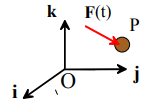By definition:

The Power developed by the force, (or the rate of work done by the force) is P = F.v . If both force and velocity are expressed in

Cartesian components, then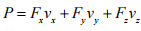Work has units of Nm/s, or `Watts’ in SI units.

The work done by the force during a time interval t0 < t<t1 is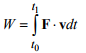The work done by the force can also be calculated by integrating the force vector along the path traveled by the force, as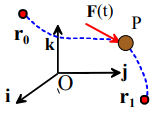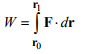where r0,r1 are the initial and final positions of the force.

Work has units of Nm in SI units, or `Joules’

A moving force can do work on a particle, or on any moving object. For example, if a force acts to stretch a spring, it is said to do work on the spring.

4.1.2 Definition of the power and work done by a concentrated moment, couple or torque

‘Concentrated moment, ‘Couple’ and `Torque’ are different names for a ‘generalized force’ that causes rotational motion without causing translational motion. These concepts are not often used to analyze motion of particles, where rotational motion is ignored – the only application might be to analyze rotational motion of a massless frame connected to one or more particles. For completeness, however, the power-work relations for moments are listed in this section and applied to some simple problems. To do this, we need briefly to discuss how rotational motion is described. This topic will be developed further in Chapter 6, where we discuss motion of rigid bodies.Definition of an angular velocity vector Visualize a spinning object, like the cube shown in the figure. The box rotates about an axis – in the example, the axis is the line connecting two cube diagonals. In addition, the object turns through some number of revolutions every minute. We would specify the angular velocity of the shaft as a vector ω, with the following properties: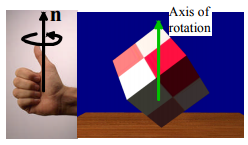1. The direction of the vector is parallel to the axis of the shaft (the axis of rotation). This direction would be specified by a unit vector n parallel to the shaft
2. There are, of course, two possible directions for n. By convention, we always choose a direction such that, when viewed in a direction parallel to n (so the vector points away from you) the shaft appears to rotate clockwise. Or conversely, if n points towards you, the shaft appears to rotate counterclockwise. (This is the `right hand screw convention’)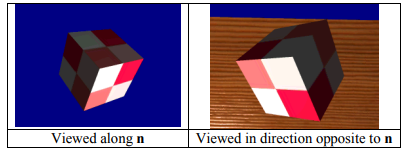3. The magnitude of the vector is the angular speed dθ / dt of the object, in radians per second. If you know the revs per minute n turned by the shaft, the number of radians per sec follows as dθ / dt  = 120πn. The magnitude of the angular velocity is often denoted by ω = dθ / dt

The angular velocity vector is then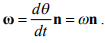Since angular velocity is a vector, it has components ω = ωxi + ωyj + ωzk in a fixed Cartesian basis.

Rate of work done by a torque or moment: If a moment M = Mxi + Myj + Mzacts on an object that rotates with angular velocity ω, the rate of work done on the object by M is

P = M.ω = Mxωx + Myωy + Mzωz

4.1.3 Simple examples of power and work calculations

Example 1: An aircraft with mass 45000 kg flying at 200 knots (102m/s) climbs at 1000ft/min. Calculate the rate of work done on the aircraft by gravity.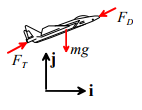The gravitational force is -mgj, and the velocity vector of the aircraft is v = vxi + vyj. The rate of work done on the aircraft is therefore

P = F.v = -mgv

Substituting numbers gives

Example 2: Calculate a formula for the work required to stretch a spring with stiffness k and unstretched length L0 from length l0 to length l1 .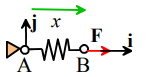The figure shows a spring that held fixed at A and is stretched in the horizontal direction by a force F = Fxi acting at B. At some instant the spring has length l0 < x < l1 . The spring force law states that the force acting on the spring at B is related to the length of the spring x by

F = Fxi = k(x - L0)i

The position vector of the force is r = xi , and therefore the work done is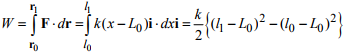Example 3: Calculate the work done by gravity on a satellite that is launched from the surface of the earth to an altitude of 250km (a typical low earth orbit).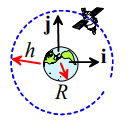Assumptions

1. The earth’s radius is 6378.145km

2. The mass of a typical satellite is 4135kg - see , e.g

3. The Gravitational parameter μ = GM = 3.986012 x 105 km3s-1 G= gravitational constant; M=mass of earth)

4. We will assume that the satellite is launched along a straight line path parallel to the i direction, starting the earths surface and extending to the altitude of the orbit. It turns out that the work done is independent of the path, but this is not obvious without more elaborate and sophisticated calculations.

Calculation:

1. The gravitational force on the satellite is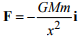2. The work done follows as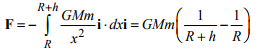3. Substituting numbers gives 9.7 x106 J (be careful with units – if you work with kilometers the work done is in N-km instead of SI units Nm)

Example 4: A Ferrari Testarossa skids to a stop over a distance of 250ft. Calculate the total work done on the car by the friction forces acting on its wheels.

Assumptions: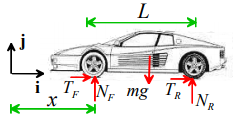1. A Ferrari Testarossa has mass 1506kg (see

2. The coefficient of friction between wheels and road is of order 0.8

3. We assume the brakes are locked so all wheels skid, and air resistance is neglected

Calculation The figure shows a free body diagram. The equation of motion for the car is

(TF + TR)i + (NR + NF - mg) j = max i

1. The vertical component of the equation of motion yields NR + NF = mg

2. The friction law shows that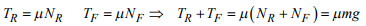3. The position vectors of the car’s front and rear wheels are rF = xi    rR = (x+L)i. The work done follows as. We suppose that the rear wheel starts at some point x0i when the brakes are applied and skids a total distance d.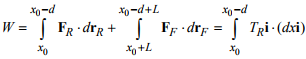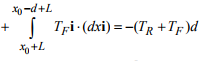4. The work done follows as W = μmg . Substituting numbers gives W = 9 x 105 J .

Example 5: The figure shows a box that is pushed up a slope by a force P. The box moves with speed v. Find a formula for the rate of work done by each of the forces acting on the box.

The figure shows a free body diagram. The force vectors are

1. Applied force P cosαi + P sinαj
2. Friction -T cos αi - T sinαj
3. Normal reaction - N sinαi + T cosαj
4. Weight -mgj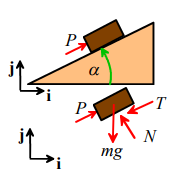The velocity vector is v cosαi + v sinαj

Evaluating the dot products F.v for each formula, and recalling that cos2α +  sin2 α = 1 gives

1. Applied force Pv
2. Friction -Tv
3. Normal reaction 0
4. Weight -mg sinα

Example 6: The table lists the experimentally measured force-v-draw data for a longbow. Calculate the total work done to draw the bow.

In this case we don’t have a function that specifies the force as a function of position; instead, we have a table of numerical values. We have to approximate the integral numerically.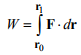Forc N Draw (cm) 0 0 40 10 90 20 140 30 180 40 220 50 270 60

To understand how to do this, remember that integrating a function can be visualized as computing the area under a curve of the function, as illustrated in the figure.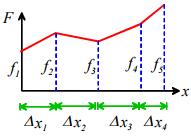We can estimate the integral by dividing the area into a series of trapezoids, as shown. Recall that the area of a trapezoid is (base x average height), so the total area of the function is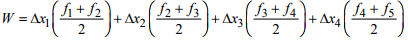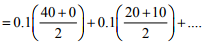You could easily do this calculation by hand – but for lazy people like me MATLAB has a convenient function called `trapz’ that does this calculation automatically. Here’s how to use it

>> draw = [0,10,20,30,40,50,60]*0.01;
>> force = [0,40,90,140,180,220,270];
>> trapz(draw,force)

ans =

80.5000

>>

So the solution is 80.5J

4.1.4 Definition of the potential energy of a conservative force

Preamble: Textbooks nearly always define the `potential energy of a force.’ Strictly speaking, we cannot define a potential energy of a single force – instead, we need to define the potential energy of a pair of forces. A force can’t exist by itself – there must always be an equal and opposite reaction force acting on a second body. In all of the discussion to be presented in this section, we implicitly assume that the reaction force is acting on a second body, which is fixed at the origin. This simplifies calculations, and makes the discussion presented here look like those given in textbooks, but you should remember that the potential energy of a force pair is always a function of the relative positions of the two forces.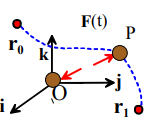With that proviso, consider a force F acting on a particle at some position r in space. Recall that the work done by a force that moves from position vector 0 r to position vector r1 is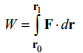In general, the work done by the force depends on the path between r0 to r1 . For some special forces, however, the work done is independent of the path. Such forces are said to be conservative.

For a force to be conservative:

The force must be a function only of its position – i.e. it can’t depend on the velocity of the force, for example.

The force vector must satisfy curl (F) = 0

Examples of conservative forces include gravity, electrostatic forces, and the forces exerted by a spring. Examples of non-conservative (or should that be liberal?) forces include friction, air resistance, and aerodynamic lift forces.

The potential energy of a conservative force is defined as the negative of the work done by the force in moving from some arbitrary initial position r0 to a new position r , i.e.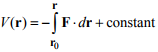The constant is arbitrary, and the negative sign is introduced by convention (it makes sure that systems try to minimize their potential energy). If there is a point where the force is zero, it is usual to put r0 at this point, and take the constant to be zero.

Note that

1. The potential energy is a scalar valued function
2. The potential energy is a function only of the position of the force. If we choose to describe position in terms of Cartesian components r = xi + yj + zk , then V (r) = V (x, y, z) .
3. The relationship between potential energy and force can also be expressed in differential form (which is often more useful for actual calculations) as F = -grad(V)

If we choose to work with Cartesian components, then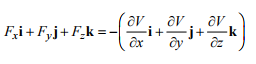Occasionally, you might have to calculate a potential energy function by integrating forces – for example, if you are interested in running a molecular dynamic simulation of a collection of atoms in a material, you will need to describe the interatomic forces in some convenient way. The interatomic forces can be estimated by doing quantum-mechanical calculations, and the results can be approximated by a suitable potential energy function. Here are a few examples showing how you can integrate forces to calculate potential energy

Offer running on EduRev: Apply code STAYHOME200 to get INR 200 off on our premium plan EduRev Infinity!

## Introduction to Dynamics and Vibrations- Notes, Videos, MCQs

20 videos|53 docs

,

,

,

,

,

,

,

,

,

,

,

,

,

,

,

,

,

,

,

,

,

,

,

,

,

,

,

;